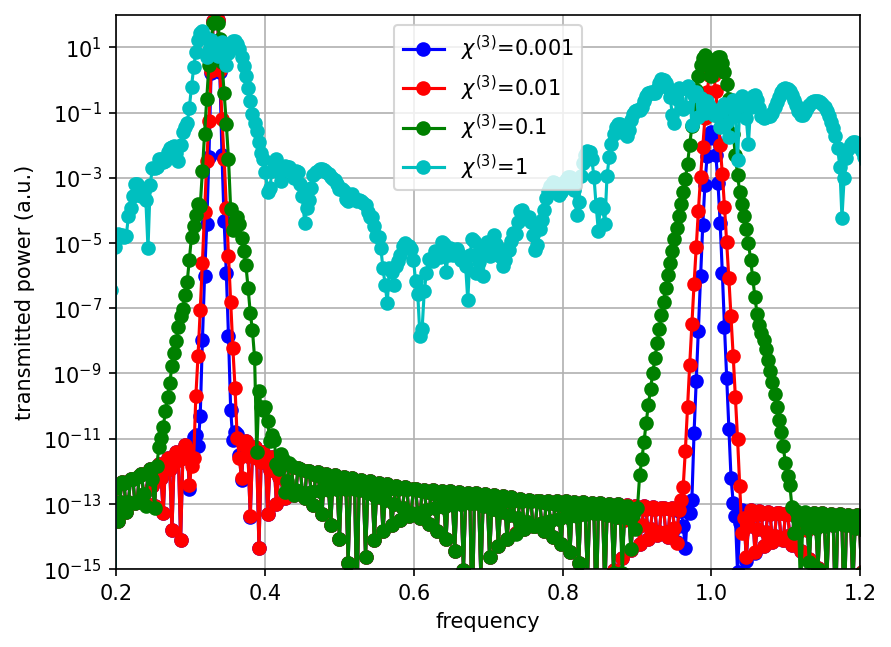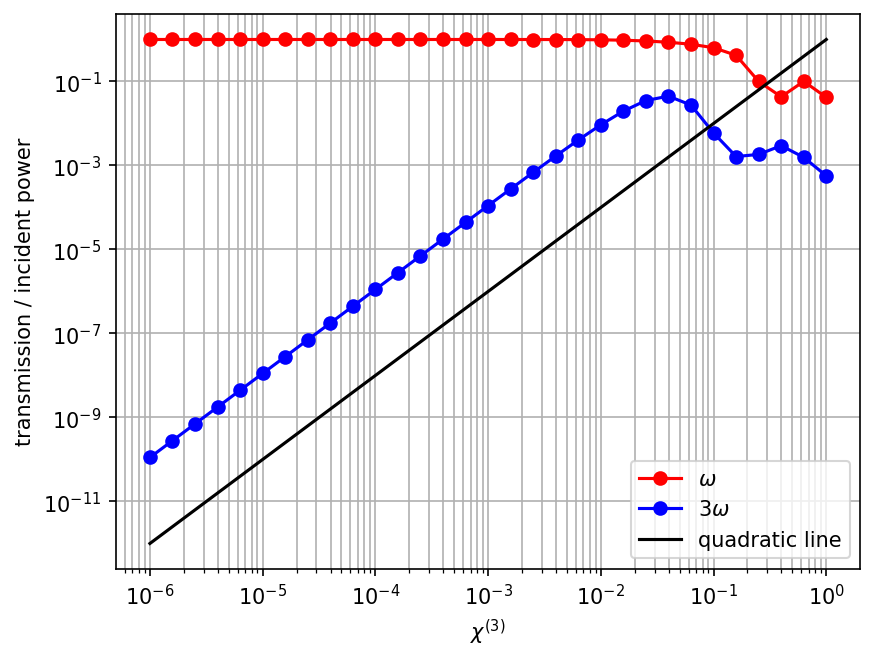# Third Harmonic Generation

In this example, we consider wave propagation through a simple 1d nonlinear medium with a non-zero Kerr susceptibility χ$^{(3)}$. See also Materials and Units and Nonlinearity. We send in a narrow-band pulse at a frequency ω, and because of the nonlinearity we also get a signal at a frequency 3ω. See also 3rd-harm-1d.ctl.

Since this is a 1d calculation, we could implement it via a 2d cell of (size S no-size no-size), specifying periodic boundary conditions in the $y$ direction. However, this is slightly inefficient since the $y$ periodic boundaries are implemented internally via extra "ghost pixels" in the $y$ direction. Instead, Meep has special support for 1d simulations in the $z$ direction. To use this, we must explicitly set dimensions to 1, and in that case we can only use $E_x$ (and $D_x$) and $H_y$ field components. This involves no loss of generality because of the symmetry of the problem.

First, as usual, we'll define some parameters of our simulation:

(define-param sz 100)          ; size of cell in z direction
(define-param fcen (/ 1 3))    ; center frequency of source
(define-param df (/ fcen 20))  ; frequency width of source
(define-param amp 1.0)         ; amplitude of source
(define-param k 1e-2)          ; Kerr susceptibility
(define-param dpml 1.0)        ; PML layer thickness


Now, to define our cell, we'll do:

(set-param! dimensions 1)
(set! geometry-lattice (make lattice (size no-size no-size sz)))
(set! pml-layers (list (make pml (thickness dpml))))
(set-param! resolution 20)


Note that this will only put PML layers at the $\pm z$ boundaries.

In this case, we're going to fill the entire computational cell with the nonlinear medium, so we don't need to use any objects. We can just use the special default-material which is ordinarily vacuum:

(set! default-material (make dielectric (index 1) (chi3 k)))


Now, our source will be a Gaussian pulse of $J_x$ just next to the $-z$ PML layer. Since this is a nonlinear calculation, we may want to play with the amplitude of the current/field, so we set the amplitude property explicitly to our parameter amp, above.

(set! sources (list
(make source
(src (make gaussian-src (frequency fcen) (fwidth df)))
(component Ex)
(center 0 0 (+ (* -0.5 sz) dpml))
(amplitude amp))))


We'll want the frequency spectrum at the $+z$ end of the computational cell. In a linear problem, we normally look at the spectrum over the same frequency range as our source, because other frequencies are zero. In this case, however, we will look from fcen/2 to 4*fcen, to be sure that we can see the third-harmonic frequency.

(define-param nfreq 400)
(define-param fmin (/ fcen 2))
(define-param fmax (* fcen 4))

(define trans ; transmitted flux
(add-flux (* 0.5 (+ fmin fmax)) (- fmax fmin) nfreq
(make flux-region (center 0 0 (- (* 0.5 sz) dpml 0.5)))))


Finally, we'll run the sources, plus additional time for the field to decay at the flux plane, and output the flux spectrum:

(run-sources+
(stop-when-fields-decayed 50 Ex (vector3 0 0 (- (* 0.5 sz) dpml 0.5)) 1e-6))

(display-fluxes trans)


In a linear calculation, we normalize the transmission against some reference spectrum, but in this case there is no obvious normalization so we will just plot the raw data for several values of k (i.e. of χ$^{(3)}$):For small values of χ$^{(3)}$, we see a peak from our source at ω=1/3 and another peak precisely at the third-harmonic frequency 3ω=1. As the χ$^{(3)}$ gets larger, frequency-mixing within the peaks causes them to broaden, and finally for χ$^{(3)}=1$ we start to see a noisy, broad-spectrum transmission due to the phenomenon of modulation instability. Notice also that at around $10^{-13}$ the data looks weird; this is probably due to our finite simulation time, imperfect absorbing boundaries, etcetera. We haven't attempted to analyze it in detail for this case.

It is also interesting to have a more detailed look at the dependence of the power at ω and 3ω as a function of χ$^{(3)}$ and the current amplitude. We could, of course, interpolate the flux spectrum above to get the desired frequencies, but it is easier just to add two more flux regions to Meep and request exactly the desired frequency components. That is, we'll add the following before run-sources+:

(define trans1 (add-flux fcen 0 1
(make flux-region (center 0 0 (- (* 0.5 sz) dpml 0.5)))))
(define trans3 (add-flux (* 3 fcen) 0 1
(make flux-region (center 0 0 (- (* 0.5 sz) dpml 0.5)))))


We could print these with more display-fluxes lines, but it is nice to print these on a single line along with χ$^{(3)}$ and the amplitude, so that we can eventually put them all into one table in our plotting program. To do this, we'll use the lower-level function (get-fluxes trans1), which returns a list of the flux values, and take the first element of the list since there is only one:

(print "harmonics:, " k ", " amp ", "
(first (get-fluxes trans1)) ", " (first (get-fluxes trans3)) "\n")


Notice how we separated everything with commas, and prefixed the line with "harmonics:" for easy grepping later.

We want to run this for a bunch of values of χ$^{(3)}$. We could write a loop in Scheme, but it is often more convenient just to use the Unix shell when we want to wrap the entire simulation in a loop. In particular, for the Bash shell, we'll just do:

 unix% (for logk in seq -6 0.2 0; do meep k="(expt 10 \$logk)" 3rd-harm-1d.ctl |grep harmonics:; done) | tee harmonics.dat


Notice how we've used the seq function to get a sequence of exponents from -6 to 0 in steps of 0.2, and how we've used a Scheme function (expt 10 x) to get $10^x$ for a logarithmic scale.

If we run the simulation with k=0, i.e. for a linear medium, we get:

harmonics:, 0, 1.0, 225.25726603587043, 5.026979706160964e-16


That is, the linear transmission is 225.25726603587043 at ω, so we'll divide by this value and plot the fractional transmission at ω and 3ω as a function of χ$^{(3)}$ on a log-log scale:As can be shown from coupled-mode theory or, equivalently, follows from Fermi's golden rule, the third-harmonic power must go as the square of χ$^{(3)}$ as long as the nonlinearity is weak (i.e. in the first Born approximation limit, where the ω source is not depleted significantly). This is precisely what we see on the above graph, where the slope of the black line indicates an exact quadratic dependence, for comparison. Once the nonlinearity gets strong enough, however, this approximation is no longer valid and the dependence is complicated.

Finally, we note that increasing the current amplitude by a factor of $F$ or the Kerr susceptibility χ$^{(3)}$ by a factor $F^3$ should generate the same third-harmonic power in the weak nonlinearity approximation. And indeed, we see:

unix% meep k=1e-3 amp=1.0 3rd-harm-1d.ctl |grep harmonics:
harmonics:, 0.001, 1.0, 225.2091048223644, 0.021498041565849526

unix% meep k=1e-6 amp=10.0 3rd-harm-1d.ctl |grep harmonics:
harmonics:, 1.0e-6, 10.0, 22525.588597389557, 0.021791784143189268


which have third-harmonic powers differing by about 1%.